2014-02-22 20:08:05 lxy201700 阅读数 5358
• ###### MATLAB图像处理

全面系统的学习MATLAB在图像处理中的应用

20342 人正在学习 去看看 魏伟

频域滤波器如小波变换，傅里叶变换，余弦变换，形态学滤波（通过膨胀和腐蚀等形态学进行去噪）。

双边，引导保边去噪。

低噪度下，拍出的图片属于泊松分布噪声，可以采用一些3D去噪算法，如BM3D算法

2018-01-06 21:24:55 zhangquan2015 阅读数 42805
• ###### MATLAB图像处理

全面系统的学习MATLAB在图像处理中的应用

20342 人正在学习 去看看 魏伟

Github个人博客：https://joeyos.github.io

## 图像去噪常用方法

### 基于离散余弦变换的图像去噪

``````%读取图像
X=rgb2gray(X);
%读取图像尺寸
[m,n]=size(X);
%给图像加噪
Xnoised=imnoise(X,'speckle',0.01);
%输出加噪图像
subplot(121);
imshow(Xnoised);
%DCT变换
Y=dct2(Xnoised);
I=zeros(m,n);
%高频屏蔽
I(1:m/3,1:n/3)=1;
Ydct=Y.*I;
%逆DCT变换
Y=uint8(idct2(Ydct));
%结果输出
subplot(122);
imshow(Y);

``````### 基于小波变换的图像去噪

``````clear;
X=rgb2gray(X);
subplot(221);
imshow(X);
title('原始图像');
% 生成含噪图像并图示
init=2055615866;
randn('seed',init);
X=double(X);
% 添加随机噪声
XX=X+8*randn(size(X));
subplot(222);
imshow(uint8(XX));
title(' 含噪图像 ');
%用小波函数coif2对图像XX进行2层
% 分解
[c,l]=wavedec2(XX,2,'coif2');
% 设置尺度向量
n=[1,2];
% 设置阈值向量 , 对高频小波系数进行阈值处理
p=[10.28,24.08];
nc=wthcoef2('h',c,l,n,p,'s');
% 图像的二维小波重构
X1=waverec2(nc,l,'coif2');
subplot(223);
imshow(uint8(X1));
%colormap(map);
title(' 第一次消噪后的图像 ');
%再次对高频小波系数进行阈值处理
mc=wthcoef2('v',nc,l,n,p,'s');
% 图像的二维小波重构
X2=waverec2(mc,l,'coif2');
subplot(224);
imshow(uint8(X2));
title(' 第二次消噪后的图像 ');
``````2019-08-27 19:03:41 qq_39594939 阅读数 190
• ###### MATLAB图像处理

全面系统的学习MATLAB在图像处理中的应用

20342 人正在学习 去看看 魏伟

## （一）傅里叶变换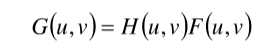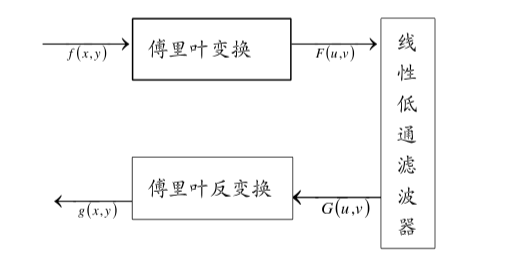np.fft.fft2()

``````import cv2
import numpy as np
from matplotlib import pyplot as plt

f=np.fft.fft2(img)
fshift=np.fft.fftshift(f)
magnitude_spectrum=20*np.log(np.abs(fshift))  #取绝对值是为了将复数变换成实数，
#取对数的目的是将数据变化到较小的范围（比如0--255）

plt.subplot(121),plt.imshow(img, cmap = 'gray')
plt.title('Input Image'), plt.xticks([]), plt.yticks([])
plt.subplot(122),plt.imshow(magnitude_spectrum, cmap = 'gray')
plt.title('Magnitude Spectrum'), plt.xticks([]), plt.yticks([])
plt.show()
``````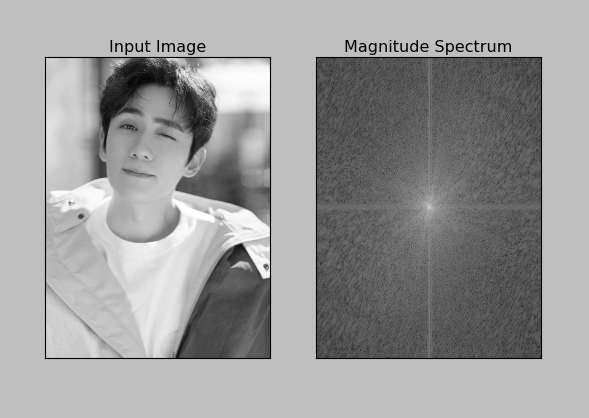``````import cv2
import numpy as np
from matplotlib import pyplot as plt

f=np.fft.fft2(img)
fshift=np.fft.fftshift(f)

ph_f=np.angle(f)
ph_fshift=np.angle(fshift)

plt.subplot(121),plt.imshow(ph_f, cmap = 'gray')
plt.title('original'), plt.xticks([]), plt.yticks([])
plt.subplot(122),plt.imshow(ph_fshift, cmap = 'gray')
plt.title('center'), plt.xticks([]), plt.yticks([])
plt.show()
``````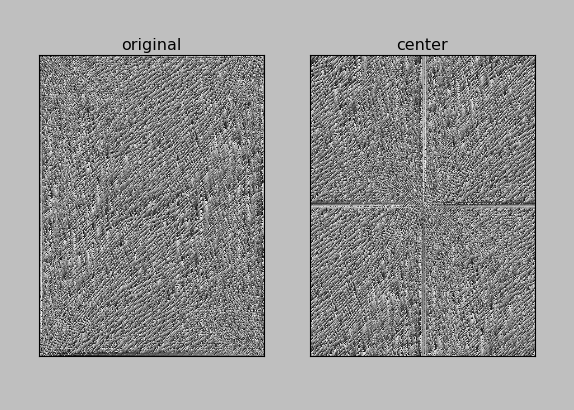1.高通滤波器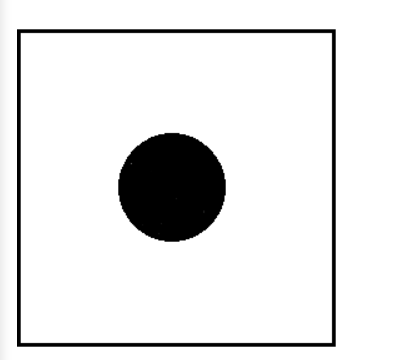``````import cv2
import numpy as np
from matplotlib import pyplot as plt
f=np.fft.fft2(img)
fshift=np.fft.fftshift(f)
magnitude_spectrum=20*np.log(np.abs(fshift))  #取绝对值是为了将复数变换成实数，
#取对数的目的是将数据变化到较小的范围（比如0--255）
rows,cols=img.shape
crow,ccol=int(rows/2),int(cols/2)

f_ishift=np.fft.ifftshift(fshift)
img_back=np.fft.ifft2(f_ishift) # 傅里叶逆变换
img_back=np.abs(img_back) # 得到的是复数会无法显示

plt.subplot(221),plt.imshow(img, cmap = 'gray')
plt.title('original'), plt.xticks([]), plt.yticks([])
plt.subplot(222),plt.imshow(img_back, cmap = 'gray')
plt.title('Image after HPF'), plt.xticks([]), plt.yticks([])
plt.show()

``````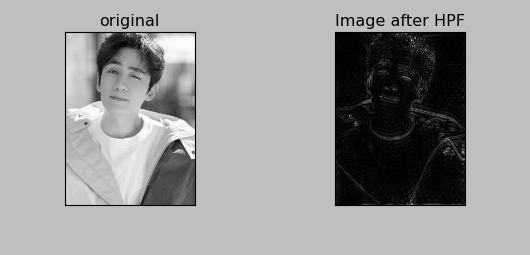2.低通滤波器

``````import cv2
import numpy as np
from matplotlib import pyplot as plt

f=np.fft.fft2(img)
fshift=np.fft.fftshift(f)
magnitude_spectrum=20*np.log(np.abs(fshift))  #取绝对值是为了将复数变换成实数，
#取对数的目的是将数据变化到较小的范围（比如0--255）

rows,cols=img.shape
crow,ccol=int(rows/2),int(cols/2)

f_ishift=np.fft.ifftshift(fshift)
img_back=np.fft.ifft2(f_ishift) # 傅里叶逆变换
img_back=np.abs(img_back) # 得到的是复数会无法显示

plt.subplot(221),plt.imshow(img, cmap = 'gray')
plt.title('original'), plt.xticks([]), plt.yticks([])
plt.subplot(222),plt.imshow(img_back, cmap = 'gray')
plt.title('Image after LPF'), plt.xticks([]), plt.yticks([])
plt.show()
``````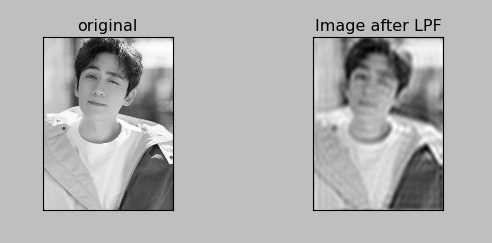3.带通滤波器

``````import cv2
import numpy as np
from matplotlib import pyplot as plt

f=np.fft.fft2(img)
fshift=np.fft.fftshift(f)

rows,cols=img.shape
crow,ccol=int(rows/2),int(cols/2)

f_ishift=np.fft.ifftshift(fshift)
img_back=np.fft.ifft2(f_ishift) # 傅里叶逆变换
img_back=np.abs(img_back) # 得到的是复数会无法显示

plt.subplot(221),plt.imshow(img, cmap = 'gray')
plt.title('original'), plt.xticks([]), plt.yticks([])
plt.subplot(222),plt.imshow(img_back, cmap = 'gray')
plt.title('Image after BPF'), plt.xticks([]), plt.yticks([])
plt.show()
``````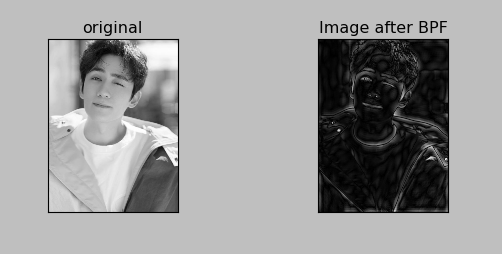## （二）基于小波变换的图像去噪技术

1.小波阈值去噪介绍

2.小波阈值去噪方法

①对原始信号进行小波分解
②对变换后的小波系数进行阈值处理，得到估计小波系数
③根据估计小波系数进行小波重构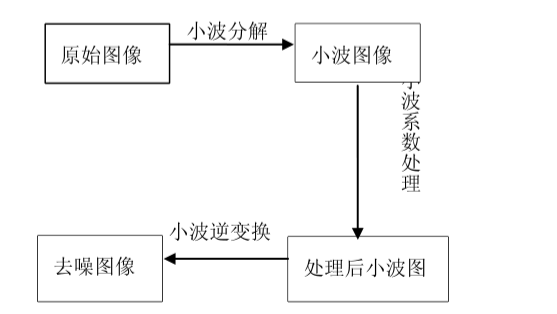3.小波阈值去噪基本问题

1. 小波基的选择：通常我们希望所选取的小波满足以下条件：正交性、高消失矩、紧支性、对称性或反对称性。但事实上具有上述性质的小波是不可能存在的，因为小波是对称或反对称的只有Haar小波，并且高消失矩与紧支性是一对矛盾，所以在应用的时候一般选取具有紧支的小波以及根据信号的特征来选取较为合适的小波。
2. 阈值的选择：直接影响去噪效果的一个重要因素就是阀值的选取，不同的阈值选取将有不同的去噪效果。目前主要有通用阈值（VisuShrink）、SureShrink阈值、Minimax阈值、BayesShrink阈值等。
3. 阈值函数的选择：阈值函数是修正小波系数的规则，不同的反之函数体现了不同的处理小波系数的策略。最常用的阈值函数有两种：一种是硬阈值函数，另一种是软阈值函数。还有一种介于软、硬阈值函数之间的Garrote函数。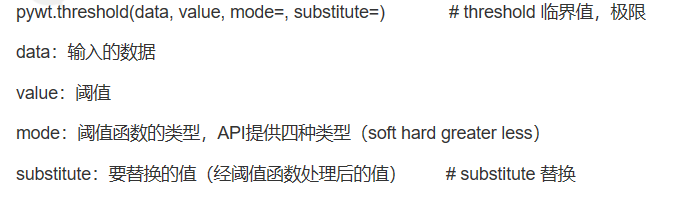``````import pywt
import numpy as np
import pandas as pd
import matplotlib.pyplot as plt
import math

# np.linspace()函数用于在指定的间隔内返回均匀间隔的数字。
data=np.linspace(1,10,10)
#[1. 2. 3. 4. 5. 6. 7. 8. 9. 10.]

# pywt.threshold(data,value,mode,substitute) mode 模式有四种，soft,hard,greater,less;substitute是替换值

data_soft=pywt.threshold(data=data,value=6,mode='soft',substitute=12)
# soft模式把小于6的值设置为12，大于等于6的值全部减6
#[12. 12. 12. 12. 12. 0. 1. 2. 3. 4.]

data_hard=pywt.threshold(data=data,value=6,mode='hard',substitute=12)
# hard模式把小于6的值设置为12，其余的值不变
#[12. 12. 12. 12. 12. 6. 7. 8. 9. 10.]

data_greater=pywt.threshold(data,6,'greater',12)
# 把小于6的值设为12，大于等于阈值的值不变
#[12. 12. 12. 12. 12. 6. 7. 8. 9. 10.]

data_less=pywt.threshold(data,6,'less',12)
# 把大于6的值设为12，小于等于阈值的值不变
#[1. 2. 3. 4. 5. 6. 12. 12. 12. 12. 12. ]
``````

4.小波去噪实验

pywt.dwt_max_level(data_len,filter_len):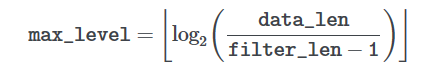pywt.wavedec(data, wavelet, mode=‘symmetric’, level=None, axis=-1)
data:输入数据，wavelet：使用的小波，level：分解级别，默认为dwt_max_len

n代表分解的级别，第一个cA_n代表的是近似分解系数，接下来的元素cD_n-cD1代表的是系数数组的详细信息

``````import pywt
import numpy as np
import pandas as pd
import matplotlib.pyplot as plt
import math

data=np.linspace(1,10,10)

# Get Data
ecg=pywt.data.ecg() # 生成心电信号
index=[]
data=[]
for i in range(len(ecg)-1):
X=float(i)
Y=float(ecg[i])
index.append(X)
data.append(Y)

# Create wavelet object and define parameters
w=pywt.Wavelet('db8') # 创建一个小波对象 选用Daubechies8小波
#  Family name:    Daubechies
#  Short name:     db
#  Filters length: 16  #滤波器长度为16
#  Orthogonal:     True  #正交
#  Biorthogonal:   True  #双正交
#  Symmetry:       asymmetric  # 对称性：不对称
#  DWT:            True  #离散小波变换
#  CWT:            False
maxlev=pywt.dwt_max_level(len(data),w.dec_len)  # 计算最大有用的分解级别（data_len,filter_len)
print("maximum level is " + str(maxlev))
threshold=0.04 # Threshold for filtering

# Decompose into wavelet components,to the level selected
coeffs=pywt.wavedec(data,'db8',level=maxlev)

plt.figure()
for i in range(1,len(coeffs)):
coeffs[i]=pywt.threshold(coeffs[i],threshold*max(coeffs[i]))  # 将噪声滤波

datarec=pywt.waverec(coeffs,'db8')  # 将信号进行小波重构

mintime=0
maxtime=mintime+len(data)+1

plt.figure()
plt.subplot(2,1,1)
plt.plot(index[mintime:maxtime],data[mintime:maxtime])
plt.xlabel('time(s)')
plt.ylabel('microvolts (uV)')
plt.title("Raw signal")
plt.subplot(2,1,2)
plt.plot(index[mintime:maxtime],datarec[mintime:maxtime-1])
plt.xlabel('time(s)')
plt.ylabel('microvolts (uV)')
plt.title("De-noised signal using wavelet techniques")

plt.tight_layout()
plt.show()
``````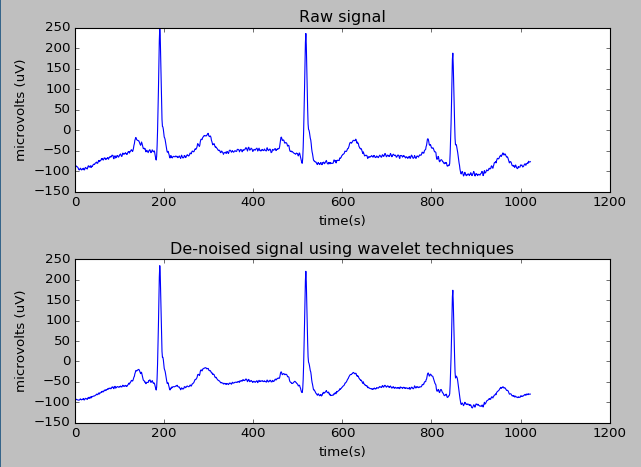Python小波变换去噪

2014-11-02 12:00:05 feifei20141029 阅读数 3165
• ###### MATLAB图像处理

全面系统的学习MATLAB在图像处理中的应用

20342 人正在学习 去看看 魏伟

1.      什么是“图像的噪声”

噪声可以理解为“妨碍人们感觉器官对所接收的信源信息理解的因素”。噪声在理论上定义为“不可预测、只能用概率统计方法来认识的随机误差”。因此，将图像噪声看成是多维随机过程是合适的，因而描述噪声的方法完全可以借用随机过程的描述，即用其概率分布函数和概率密度分布函数。但在很多情况下，这样的描述方法是很复杂的，甚至是不可能的，而实际应用往往也不必要。通常是用其数字特征，即均值方差、相关函数等，因为这些数字特征都可以从某些方面反映出噪声的特征。

①  外部噪声，即指系统外部干扰以电磁波的形式进入系统内部而引起的噪声，如电气设备、天体放电现象等引起的噪声。

②  内部噪声：一般又可以分为以下四种。

Ø  由光和电的基本性质所引起的噪声。

Ø  电器的机械运动产生的噪声，如各种接头因抖动引起电流变化所产生的噪声，磁头、磁带等运动。

Ø  器材材料本身引起的噪声，如正片和负片的表面颗粒性和磁带磁盘表面缺陷所产生的噪声。随着材料科学的发展，这些噪声有望不断减少，但目前还是不可避免的。

Ø  系统内部设备电路所引起的噪声，如电源引入的交流噪声，偏转系统所引起的噪声等。

图像噪声从统计理论观点可以分为平稳和非平稳噪声两种：统计特性不随时间变化的噪声称为平稳噪声；统计特性随时间变化而变化的噪声称为非平稳噪声。

2.      图像去噪常用方法

图像的去噪处理方法可以分为空间域法和变换域法两大类。前者是在原图像上直接进行数据运算，对像素的灰度值进行处理。后者是在图像的变换域上进行处理，对变换后的系数进行相应的处理，然后进行反变换达到图像去噪的目的。

2.1  基于离散余弦变换的图像去噪

基于离散余弦变换对图像的噪声抑制原理如下：一般而言，我们认为图像的噪声在离散余弦变换结果中处在其高频部分，而高频部分的幅值一般很小，利用这一性质，就很容易实现图像的噪声抑制。当然，这会同时失去图像的部分细节。Fig.1  添加噪声的图像和去噪后的图像

2.2  基于小波变换的图像去噪

小波去噪是小波变换较为成功的一类应用，其去噪的基本思路如下：

因此，利用小波变换在去除噪声时可提取并保存对视觉起主要作用的边缘信息。而传统的基于傅里叶变换去噪方法在去除噪声和边沿保持上存在着矛盾，原因是傅里叶变换方法在时域不能局部化，难以检测到局域突变信号，在去除噪声的同时，也损失了图像边沿信息。由此可见，与基于傅里叶变换去噪方法相比，基于小波变换去噪方法具有明显的优越性。

Donoho提出的小波阈值去噪方法的基本思想是：当小波系数小于某个临界阈值时，认为这时的小波系数主要是由噪声引起的，予以舍弃；当小波系数大于这个临界阈值时，认为这时的小波系数主要是由信号引起，那么就把这部分小波系数直接保留下来（硬阈值方法），或者按某一个固定量向零收缩（软阈值方法），然后用新的小波系数进行小波重构得到去噪后的信号。实现步骤如下：

①  先对含噪声的信号做小波变换，得到一组小波分解系数；

②  通过对分解得到的小波系数进行阈值处理，得到估计小波系数；

③  利用估计小波系数进行小波重构，得到估计信号，即为去噪后的信号。

阈值函数关系着重构信号的连续性和精度，对小波去噪的效果有很大影响。目前，阈值的选择主要分为硬阈值和软阈值两种处理方式。其中，软阈值处理是将信号的绝对值与 阈值进行比较，当数据的绝对值小于或等于阈值时，令其为零；大于阈值的数据点则向零收缩，变为该点值与阈值之差。而硬阈值处理时将信号的绝对值阈值进行比较，小于或等于阈值的点变为零，大于阈值的点不变。硬阈值函数的不连续性使消噪后的信号仍然含有明显的噪声；采用软阈值方法虽然连续性好，但估计小波系数与含噪声信号的小波系数之间存在恒定的偏差。Fig.2  添加噪声的图像和小波水平垂直去噪后的图像

2017-03-09 15:09:48 mingtian715 阅读数 60064
• ###### MATLAB图像处理

全面系统的学习MATLAB在图像处理中的应用

20342 人正在学习 去看看 魏伟

图像去噪是信号处理的一个经典问题，传统的去噪方法多采用平均或线性方法进行，常用的是维纳滤波，但是去噪效果不太好（维纳滤波在图像复原中的作用）。随着小波理论的日益完善，其以自身良好的时频特性在图像去噪领域受到越来越多的关注，开辟了用非线性方法去噪的先河。具体来说，小波能够去噪主要得益于小波变换有如下特点：
（1）低熵性。小波系数的稀疏分布，使图像变换后的熵降低。意思是对信号（即图像）进行分解后，有更多小波基系数趋于0（噪声），而信号主要部分多集中于某些小波基，采用阈值去噪可以更好的保留原始信号。
（2）多分辨率特性。由于采用了多分辨方法，所以可以非常好地刻画信号的非平稳性，如突变和断点等（例如0-1突变是傅里叶变化无法合理表示的），可以在不同分辨率下根据信号和噪声的分布来消除噪声。
（3）去相关性。小波变换可对信号去相关，且噪声在变换后有白化趋势，所以小波域比时域更利于去噪。
（4）基函数选择灵活。小波变换可灵活选择基函数，也可根据信号特点和去噪要求选择多带小波和小波包等（小波包对高频信号再次分解，可提高时频分辨率），对不同场合，选择不同小波基函数。

（1）基于小波变换模极大值去噪（信号与噪声模极大值在小波变换下会呈现不同变化趋势）
（2）基于相邻尺度小波系数相关性去噪（噪声在小波变换的各尺度间无明显相关性，信号则相反）
（3）基于小波变换阈值去噪

（1）硬阈值（2）软阈值（1）二维信号的小波分解。选择一个小波和小波分解的层次N，然后计算信号s到第N层的分解。
（2）对高频系数进行阈值量化。对于从1~N的每一层，选择一个阈值，并对这一层的高频系数进行软阈值量化处理。
（3）二维小波重构。根据小波分解的第N层的低频系数和经过修改的从第一层到第N的各层高频系数，计算二维信号的小波重构

Matlab函数介绍
（1）wavedec2函数

[C,S] = wavedec2(X,N,'wname'):用小波函数wname对信号X在尺度N上的二维分解，N是严格正整数。

（2）wrcoef2函数

X = wrcoef2('type',C,S,'wname',N):用指定的小波函数wname进行N尺度重构。当type = 'a'时，仅对信号的低频部分进行重构，此时N可以为0;当type = 'h'(或'v'/'d')时，对信号（水平、垂直、对角）的高频进行重构，N为严格正整数。

（3）wthcoef2函数

NC = wthcoef2('type',C,S,N,T,SORH):返回经过小波分解结构[C,S]进行处理后的新的小波分解向量NC，[NC,S]即构成一个新的小波分解结构。N是一个包含高频尺度的向量，T是相应的阈值，且N和T长度须相等。返回'type'（水平、垂直、对角线）方向的小波分解向量NC。参数SORH用来对阈值方式进行选择，当SORH = 's'时，为软阈值，当SORH = 'h'时，为硬阈值。

```clear all;
subplot(2,2,1);image(X);
colormap(map);
xlabel('(a)原始图像');
axis square
%产生含噪声图像
init = 2055615866;
randn('seed',init);
x = X + 50*randn(size(X));
subplot(2,2,2);image(x);
colormap(map);
xlabel('(b)含噪声图像');
axis square
%下面进行图像的去噪处理
%用小波函数coif3对x进行2层小波分解
[c,s] = wavedec2(x,2,'coif3');
%提取小波分解中第一层的低频图像，即实现了低通滤波去噪
%设置尺度向量
n = [1,2];
%设置阈值向量p
p = [10.12,23.28];
%对三个方向高频系数进行阈值处理
nc = wthcoef2('h',c,s,n,p,'s');
nc = wthcoef2('v',nc,s,n,p,'s');
nc = wthcoef2('d',nc,s,n,p,'s');
%对新的小波分解结构[c,s]进行重构
x1 = waverec2(nc,s,'coif3');
subplot(2,2,3);image(x1);
colormap(map);
xlabel('(c)第一次去噪图像');
axis square
%对nc再次进行滤波去噪
xx = wthcoef2('v',nc,s,n,p,'s');
x2 = waverec2(xx,s,'coif3');
subplot(2,2,4);image(x2);
colormap(map);
xlabel('(d)第二次去噪图像');
axis square
```